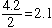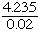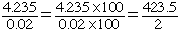Hello--I teach math and my class and I were discussing the dividing of decimals. I explained that if there is a decimal in the divisor, it needs to be moved and so does the decimal in the dividend. My question is why is it necessary to move the decimal point in the divisor before dividing? thanks---Alex Hi Alex, You "move the decimals", of course, in order to know where to place the decimal in the quotient. If the divisor is an integer then you don't need to move the decimals and the division is straightforward,If the divisor is not an integer then the technique is to replace the division by an equivalent problem where the divisor is an integer. Thus formultiply the numerator and denominator by 100 to get the equivalent fractionand then divide. Cheers, Penny Go to Math Central